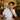# Implement K-Means clustering from scratch ✨

October 23, 2020

This Youtube lecture really helped me to get started. Then I modified & updated class methods for my own purpose.

## Intro

I will try to explain the pseudocode first, and go into implementation details with python after.

## Pseudocode

for simplicity, I will consider each sample data is 2-dimensional. (x,y)

1. Decide your K(2,3 or etc) to get started.
2. Position K number of centroids in random position.
3. Loop over all sample data, and get distances from all K number of centroids.
4. Label each sample data with cluster index, based on the shorted distances from each sample point to all centroids.
5. Update(Center again) all centroids position based on the labeld sample data.
6. Repeat step 3-5 until the centroids position updates are no more significant (converges)

Now Let’s see how we could implement in python based on the pseudocode

## Implementation

``````import numpy as np

np.random.seed(42)``````
• numpy library will be used.
• with random seed, you can fix the random ouput for each run.

Let’s define a Class.

``````class KMeans():
self.K = K
self.max_iters = max_iters
self.plot_steps = plot_steps

# create initial clusters based on K
self.clusters = [[] for _ in range(self.K)]
# initial centroids
self.centroids = []``````
• max_iters: we should have the maximum iteration to avoid inifite centroid position updates.
• plot_steps: This is an optional. With this boolean, we could plot the scatter figures step by step.

### public `FIT` method

This is a main `fit` method. This will train the train sample data to get the K-Means clustering Model Ready.

``````def fit(self, X_train):
"""Summary or Description of the Function

Parameters:
X_train ([np.array,]): X input for training

Returns:
None

"""
self.X_train = X_train
self.n_samples, self.n_features = X_train.shape

# initialize
random_sample_idxs = np.random.choice(self.n_samples, self.K, replace=False)
self.centroids = [self.X_train[idx] for idx in random_sample_idxs]

# Optimize clusters
for _ in range(self.max_iters):
# create clusters for each iteration
self.clusters = self._create_clusters(self.centroids)

if self.plot_steps:
self.plot()

# prepare old and new calculated centroids
centroids_old = self.centroids
self.centroids = self._update_centroids(self.clusters)

# check if converges
if self._is_converged(centroids_old, self.centroids):
break

if self.plot_steps:
self.plot()
self.plot()``````
1. We choose initial random centroid positions.
2. Start the loop with max iterations.
3. Create clusters based on the centroid positions.
4. Keep old centroid positions.
5. Update the current centroid positions
6. If the centroid position is converged, which means, no more updates, the loop stops.

### private methods

Let’s find more about private methods used in `fit` method.

``````def _create_clusters(self, centroids):
# first, create an empty cluster list of lists based on K
clusters = [[] for _ in range(self.K)]

# loop over X_train
for idx, sample in enumerate(self.X_train):
centroid_idx = self._get_nearest_centroid(sample, centroids)
clusters[centroid_idx].append(sample)

return clusters``````
• with this `_create_clusters` method, we can label clusters for each given X train data.
• In clusters (list of list data type), each cluster is grouped.
• Each cluster group have (probably) many sample data.
``````def _update_centroids(self, clusters):
# assign mean value of clusters to centroids
centroids = np.zeros((self.K, self.n_features))
for cluster_idx, cluster in enumerate(clusters):
cluster_mean = np.mean(cluster, axis=0)
centroids[cluster_idx] = cluster_mean
return centroids``````
• This will simply update centroids.
``````def _is_converged(self, centroids_old, centroids_current):
# distances between each old and new centroids, fol all centroids
distances = [get_distance(centroids_old[i], centroids_current[i]) for i in range(self.K)]
return sum(distances) == 0``````
• return boolean, if we need another centroids position update.

### public `predict` method

Now this method will help you to predict new data cluster label.

``````def predict(self, X_test):
return self._get_cluster_labels(X_test)``````
• I created `_get_cluster_labesl` in separate, because it was more explicit, and perhaps we could do more than getting cluster labels with `predict` method in the future.
``````def _get_cluster_labels(self, X_test):
# each sample will get the label of the cluster it was assigned to
n_samples, features = X_test.shape

# get empty labels
labels = np.empty(n_samples)

# we calculate closest centroid and append the centroid idx to labels
for sample_idx, sample in enumerate(X_test):
centroid_idx = self._get_nearest_centroid(sample, self.centroids)
labels[sample_idx] = centroid_idx
return labels``````
• This will get new label(y) data based on the nearest centroid for each sample.
• The indices are matched with `X_test`.
``````def _get_nearest_centroid(self, sample, centroids):
# first get distances for given sample from each centroid
distances = [get_distance(sample, point) for point in centroids]

# get centroid index where distance is the smallest
closest_index = np.argmin(distances)
return closest_index``````
• get all distances and return the closest
• `np.argmin` will return the indices of the minimum values along an axis.
``````def _get_distance(self, x1, x2):
return np.sqrt(np.sum((x1 - x2)**2))``````
• get Eculidian Distance.
``````def plot(self):
fig, ax = plt.subplots(figsize=(12, 8))

for i, cluster in enumerate(self.clusters):
ax.scatter(*zip(*cluster))

for point in self.centroids:
ax.scatter(*point, marker="x", color='black', linewidth=2)

plt.show()``````
• This will simply draw scatter plot with centroids.

## Compare My own implementation vs sklearn library

for the test code setup, see HERE

### 1. My own implementation### 2. sklearn library version implementationAs we can see both results, both are not so good, and thererfore it is hard to see which one does better performance. For both ‘my own implementation’ and ‘sklearn version implementation’ we used `random` initial centroids positions. For ‘sklearn version’, I tried with `k-means++` initial centroid position option, but the result gave me no improvement at all.

## Future Todos

1. Apply Standardization for both my implementation and sklearn library implementation.
2. Find the dataset working well with sklearn library, and apply my own implementation.
3. try diffent Ks, or find the best K method

## Apply Standardaziation

After applying StandardScalar, here are better results. Therefore, future todos 1 and 2 are not

### 1. My own implementation### 2. sklearn library version implementationIt is obvious that sklearn version is now doing its job. K-4 clustering is clear now, however my own implementation is still not working well.

## Code Repository

You can find the full working code in this GitHub LinkWelcome to Johnny Ilmo Koo's blog

...